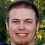## Overview

Java was designed with the principle that you shouldn't need to know the size of an object. There are times when you really would like to know and want to avoid the guess work.

## Measuring how much memory an object uses

There are three factors which make measuring how much an object uses difficult.
• The TLAB allocates blocks of memory to a thread.  This means small amount of memory don't appear to reduce the free memory. If you do this repeatedly, you will see a block of free memory be used. The way around this is to turn off the TLAB. -XX:-UseTLAB
• A GC can occur while you are creating your object. This will result in more free memory at the end than when you started. I ignore any negative sizes in this test ;)
• Other threads in the system could use memory at the same time. I perform multiple test and take the median, which removes any outliers.

## Size of objects in a 32-bit JVM

Running this SizeofTest, with on 32-bit Sun/Oracle Java 6 update 26, -XX:-UseTLAB I get

```The average size of an int is 4.0 bytes
The average size of an Object is 8.0 bytes
The average size of an Integer is 16.0 bytes
The average size of a Long is 16.0 bytes
The average size of an AtomicReference is 16.0 bytes
The average size of an SimpleEntry(Map.Entry) is 16.0 bytes
The average size of a DateTime is 24.0 bytes
The average size of a Calendar is 424.0 bytes
The average size of an Exception is 400.0 bytes
The average size of a bit in a BitSet is 0.125 bytes
```

Looking a the size of Long confirms the size of header/Object being 8 bytes.

## Size of objects with 32-bit references

Running this SizeofTest, with 32-bit references On Sun/Oracle Java 6 update 26, -XX:+UseCompressedOops -XX:-UseTLAB I get
```The average size of an int is 4.0 bytes
The average size of an Object is 16.0 bytes
The average size of an Integer is 16.0 bytes
The average size of a Long is 24.0 bytes
The average size of an AtomicReference is 16.0 bytes
The average size of an SimpleEntry(Map.Entry) is 24.0 bytes
The average size of a DateTime is 24.0 bytes
The average size of a Calendar is 448.0 bytes
The average size of an Exception is 440.0 bytes
The average size of a bit in a BitSet is 0.125 bytes
```

Objects are 8-byte aligned on this JVM, and you could conclude from the size of an Integer that the header is 12-bytes in size.

## Size of objects with 64-bit references

Running the same test with 64-bit references. i.e. -XX:-UseCompressedOops -XX:-UseTLAB
```The average size of an int is 4.0 bytes
The average size of an Object is 16.0 bytes
The average size of an Integer is 24.0 bytes
The average size of a Long is 24.0 bytes
The average size of an AtomicReference is 24.0 bytes
The average size of an SimpleEntry(Map.Entry) is 32.0 bytes
The average size of a DateTime is 32.0 bytes
The average size of a Calendar is 544.0 bytes
The average size of an Exception is 648.0 bytes
The average size of a bit in a BitSet is 0.125 bytes
```
From looking at the size of a Long, confirms the size of the header/Object is 16 bytes in length.

## The code for the SizeofUtil

The code for the SizeofUtil is here

1.Thanks. I wanted such an approach.

2.As always the Calendar object size is sore to the eye... use jodatime DateTime instead! In our date heavy app it cut our memory use from approx 500MB to 200MB

3.@48, I have added sizes for Joda's DateTime and it is much smaller. ;)

4.Hi Peter,

I just found your blog and I'm enjoying going through the archives!

There is an easier way to get arbitrary object sizes that is actually useful in a "real" application: java.lang.instrument.Instrumentation.getObjectSize. I've written a utility that fleshes that out a little here: https://github.com/jbellis/jamm

5.@Jonathan, It is easier in some ways, provided you can add it as an agent to the command line.

6.Thanks for the detailed information. and also most of the times both 32 bit and 64 bit works as these expect in some cases where if there are any machine specific calls are called. 32 bit takes 4 bytes long,64 bit takes 8 bytes of size, so 32 bit takes less size compared to 64 bit machines.
To find out the 32 bit or 64 bit version 32 bit or 64 bit

7.What is the avg size of an Enum field?

8.Since an Enum is a full-blown class, would that enum field take up as much memory as an Object reference (so, on a 64-bit system, that would be 8 bytes?) ?